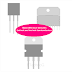The main difference between Extrinsic and Intrinsic Semiconductors is that Extrinsic semiconductor is not pure, it has some impurities added manually by dipping whereas intrinsic semiconductor material is pure, there are no impurities. A semiconductor is a type of material whose electrical current conduction properties vary between a conductive material and an insulating material. The current conduction property of a semiconductor is also nonlinear. But this property is very useful in electronics engineering. In fact, without semiconductor material, there is no existence of electronics engineering. Extrinsic and Intrinsic semiconductors are the two main types of the semiconductor.

## Intrinsic Semiconductor

An intrinsic Semiconductor is a pure form of semiconductor. It is also known as Undopped Semiconductor or i-type semiconductor. As there are no impurities, so the charge carrier can be determined by the properties of the material itself. In an intrinsic semiconductor, the number of electrons in its conduction band is equal to the holes in its valance band. This type of semiconductor conducts the electrical current with the help of electron excitation or crystallographic defects.

Intrinsic Semiconductors also have two types - indirect bandgap and direct bandgap. An indirect bandgap is that where the increase of energy in the valance band is not equal to the decreasing the energy in the conduction band. On the other hand, the direct bandgap is that where the increase of energy in the valance band is equal to the decrease of energy in the conduction band.

Examples of Intrinsic Semiconductors are Pure Silicon, Germanium, etc.

## Extrinsic Semiconductor

An extrinsic semiconductor is an impure material. It is made from intrinsic semiconductors only. During its manufacturing, a chemical or a trace element called a doping agent is mixed or dropped with the intrinsic semiconductor. It is done because to change the electrical property of the material. According to the type of dopping agent, extrinsic semiconductors are also divided into two types - P-Type Semiconductor and N-Type Semiconductor.

P means positive or hole. A P-Type semiconductor is made by the dopping of electron acceptor atoms. In the P-Type semiconductor, the majority of carriers are holes. N means negative or electrons. An N-Type semiconductor is made by the dopping electron donor atoms. In the N-Type semiconductor, the majority of carriers are electrons.

P-Type and N-Type semiconductors are examples of Extrinsic Semiconductors.

## Extrinsic Semiconductor VS Intrinsic Semiconductor

1. Extrinsic semiconductors are impure whereas Intrinsic semiconductors are pure.

2. Electrical Conductivity properties of the Extrinsic semiconductor are very poor than intrinsic semiconductor.

3. The current conduction in extrinsic semiconductors happens due to the movement of electrons and holes between valance and conduction band whereas the current conduction in an intrinsic semiconductor happens due to the excitation of the electrons or crystallographic defects.

4. The current conduction property of an extrinsic semiconductor depends upon both temperature and the dopping agent whereas the current conduction property of the intrinsic semiconductor depends upon the temperature only.

5. Energy response of the extrinsic semiconductor is volatile whereas the energy response of the intrinsic semiconductor is smooth.

Main Difference between Extrinsic and Intrinsic SemiconductorsReviewed by Author on November 05, 2021 Rating: 5